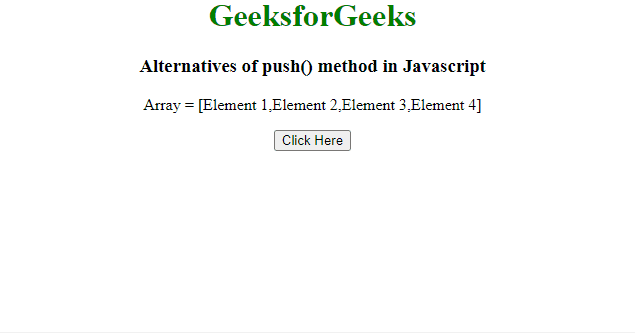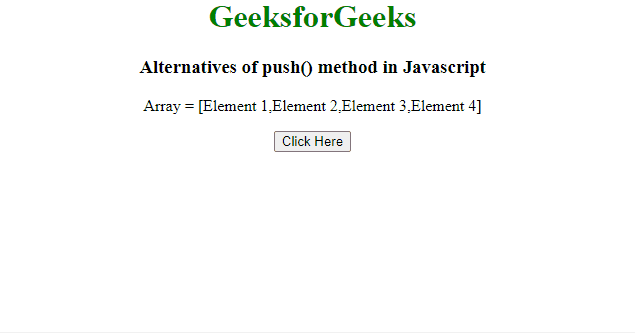# Alternatives of push() method in JavaScript

The task is to perform a push operation without using the push() method with the help of JavaScript. There are two approaches that are discussed below.

Approach 1: Use the length property to insert the element at the end of the array.

Example: This example implements the above approach.

## html

 `<``body` `style``=``"text-align: center;"``> ` `    ``<``h1` `style``=``"color: green;"``> ` `        ``GeeksforGeeks ` `    `` ` `    ``<``h3``> ` `        ``Alternatives of push() method in Javascript ` `    `` ` `    ``<``p` `id``=``"GFG_UP"``> ` `    ``<``button` `onclick``=``"myGFG()"``> ` `        ``Click Here ` `    `` ` `    ``<``p` `id``=``"GFG_DOWN"``> ` `    ``<``script``> ` `        ``var arr = ["Element 1", "Element 2", ` `                    ``"Element 3", "Element 4"]; ` `        ``var up = document.getElementById("GFG_UP"); ` `        ``up.innerHTML = "Array = [" + arr + "]"; ` `        ``var element = "Element x"; ` `        ``var down = document.getElementById("GFG_DOWN"); ` `        ``function myGFG() { ` `        ``arr[arr.length] = element; ` `        ``down.innerHTML = "Elements of array = [" + arr + "]"; ` `                ``} ` `    `` ` ``

Output:Alternatives of push() method in JavaScript

Approach 2 Use the [] notation to insert the element at the end of the array.

Example: This example implements the above approach.

## html

 `<``body` `style``=``"text-align: center;"``> ` `    ``<``h1` `style``=``"color: green;"``> ` `        ``GeeksforGeeks ` `    `` ` `    ``<``h3``> ` `        ``Alternatives of push() method in Javascript ` `    `` ` `    ``<``p` `id``=``"GFG_UP"``> ` `    ``<``button` `onclick``=``"myGFG()"``> ` `        ``Click Here ` `    `` ` `    ``<``p` `id``=``"GFG_DOWN"``> ` `    ``<``script``> ` `        ``var arr = ["Element 1", "Element 2", ` `                    ``"Element 3", "Element 4"]; ` `        ``var up = document.getElementById("GFG_UP"); ` `        ``up.innerHTML = "Array = [" + arr + "]"; ` `        ``var element = "Element x"; ` `        ``var down = document.getElementById("GFG_DOWN"); ` `        ``function myGFG() { ` `        ``arr = [arr, element]; ` `        ``down.innerHTML = "Elements of array = [" + arr + "]"; ` `                    ``} ` `    `` ` ``

Output:Alternatives of push() method in JavaScript

Whether you're preparing for your first job interview or aiming to upskill in this ever-evolving tech landscape, GeeksforGeeks Courses are your key to success. We provide top-quality content at affordable prices, all geared towards accelerating your growth in a time-bound manner. Join the millions we've already empowered, and we're here to do the same for you. Don't miss out - check it out now!